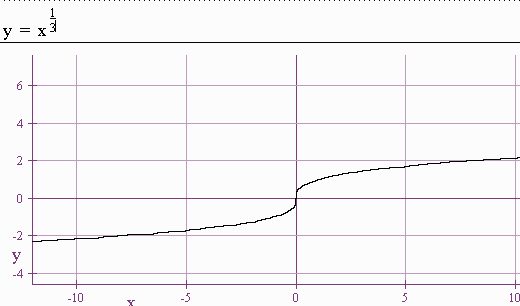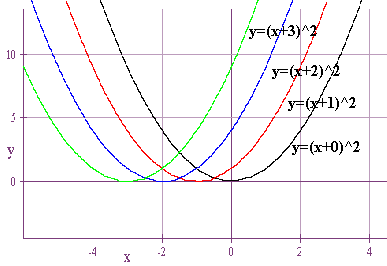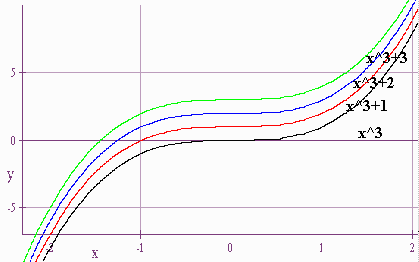$$\newcommand{\id}{\mathrm{id}}$$ $$\newcommand{\Span}{\mathrm{span}}$$ $$\newcommand{\kernel}{\mathrm{null}\,}$$ $$\newcommand{\range}{\mathrm{range}\,}$$ $$\newcommand{\RealPart}{\mathrm{Re}}$$ $$\newcommand{\ImaginaryPart}{\mathrm{Im}}$$ $$\newcommand{\Argument}{\mathrm{Arg}}$$ $$\newcommand{\norm}{\| #1 \|}$$ $$\newcommand{\inner}{\langle #1, #2 \rangle}$$ $$\newcommand{\Span}{\mathrm{span}}$$

# 1.3: Shifting and Reflecting

$$\newcommand{\vecs}{\overset { \rightharpoonup} {\mathbf{#1}} }$$ $$\newcommand{\vecd}{\overset{-\!-\!\rightharpoonup}{\vphantom{a}\smash {#1}}}$$$$\newcommand{\id}{\mathrm{id}}$$ $$\newcommand{\Span}{\mathrm{span}}$$ $$\newcommand{\kernel}{\mathrm{null}\,}$$ $$\newcommand{\range}{\mathrm{range}\,}$$ $$\newcommand{\RealPart}{\mathrm{Re}}$$ $$\newcommand{\ImaginaryPart}{\mathrm{Im}}$$ $$\newcommand{\Argument}{\mathrm{Arg}}$$ $$\newcommand{\norm}{\| #1 \|}$$ $$\newcommand{\inner}{\langle #1, #2 \rangle}$$ $$\newcommand{\Span}{\mathrm{span}}$$ $$\newcommand{\id}{\mathrm{id}}$$ $$\newcommand{\Span}{\mathrm{span}}$$ $$\newcommand{\kernel}{\mathrm{null}\,}$$ $$\newcommand{\range}{\mathrm{range}\,}$$ $$\newcommand{\RealPart}{\mathrm{Re}}$$ $$\newcommand{\ImaginaryPart}{\mathrm{Im}}$$ $$\newcommand{\Argument}{\mathrm{Arg}}$$ $$\newcommand{\norm}{\| #1 \|}$$ $$\newcommand{\inner}{\langle #1, #2 \rangle}$$ $$\newcommand{\Span}{\mathrm{span}}$$

## 1. Six Basic Functions

Below are six basic functions:

1.2.3.4.5.6.Memorize the shapes of these functions.

## 2. Horizontal Shifting

Consider the graphs$$y =$$

• $$(x+0)^2$$
• $$(x+1)^2$$
• $$(x+2)^2$$
• $$(x+3)^2$$
##### Exercise

Use the list features of a calculator to sketch the graph of

$$y = \dfrac{1}{ [x - \{0,1,2,3\}] }$$

##### Horizontal Shifting Rules
• Rule 1: $$f(x - a) = f(x)$$ shifted $$a$$ units to the right.
• Rule 2: $$f(x + a) = f(x)$$ shifted $$a$$ units to the left.

## 3. Vertical Shifting

Consider the graphs$$y =$$

• $$x^3$$
• $$x^3+ 1$$
• $$x^3 + 2$$
• $$x^3 + 3$$
##### Exercise

Use the list features of a calculator to sketch the graph of

$$y = x^3 - \{0,1,2,3\}$$

##### Vertical Shifting Rules
• Rule 3: $$f(x ) + a = f(x)$$ shifted a units up.
• Rule 4: $$f(x) - a = f(x)$$ shifted a units down.

## 4. Reflecting About the x-axis

Consider the graphs of

$$y = x^2$$ and $$y = -x^2$$.##### x-Axis Reflection Rule

Rule 5: $$-f(x) = f(x)$$ reflected about the x-axis.

## 5. Reflecting About the y-axis

##### Exercise

Use the calculator to graph

$$y=\sqrt{x}$$

and
$$y=\sqrt{-x}$$

##### y-Axis Reflection Rule

Rule 6: $$f(-x ) = f(x)$$ reflected about the y-axis.

## 6. Stretching and Compressing

##### Exercise

Graph the following:

$$y = \{1,2,3,4\}x^3$$

$$y = {1/2,1/3,1/4,1/5}x^3$$

##### Stretching and Compression rules:
• Rule 7: $$cf(x ) = f(x)$$ (for $$c > 1$$) stretched vertically.
• Rule 8: $$cf(x ) = f(x)$$ (for $$c < 1$$) compressed vertically.
##### Exercise

Graph the following

1. $$y = x^2 - 10$$
2. $$y = \sqrt{x - 2}$$
3. $$y = -|x - 5| + 3$$

We will do some examples (including the graph of the winnings for the gambler and for the casino).

## 7. Increasing and Decreasing Functions

##### Definition

A function is called increasing if as an object moves from left to right, it is moving upwards along the graph. Or equivalently,

If $$x < y$$, then $$f(x) < f(y)$$.##### Example 1

The curve

$y = x^2$

is increasing on $$(0,\infty)$$ and decreasing on $$(-\infty,0)$$.